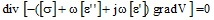# Steady State AC Electric: solved equations

## Introduction

In a Steady state AC Electric application the equations used for computation are:

• the corresponding Maxwell's equations for an electrical system, and
• the constitutive equation that characterizes the dielectric materials

The conditions of computation of a Steady state AC Electric application are the following:

• the computation concerns the D and E fields; the B and H fields are not computed. The equations of the electric fields E, D and of the magnetic fields B, H are decoupled.
• the fields are harmonic time dependent of the pulsation ω. In the complex form of the equations, the operator ∂/∂t is replaced by the multiplicator

## Equations and conditions

In the previously defined conditions of computation, the equations in complex form are summarized as follows: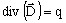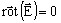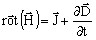⇒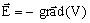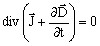E : electric field strength (in V/m) D : electric flux density (C/m2) V : electric potential (in V) J : current density (in A/m2)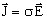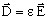⇒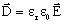σ : conductivity (in S)εr : relative permittivity ε0 : vacuum permittivity (in F/m)

Reminder about the differential operators: The curl divergence of a field is always null: div [rot (Field)] = 0.

## Solved equation

The second order equation solved by the finite elements method in Flux in case of a Steady state AC Electric application is the following: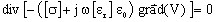where:

• [σ] is the tensor of the conductivity of the medium (in Siemens),
• r] is the tensor of the relative permittivity of dielectric materials
• ε0 is the vacuum permittivity; ε0 = 1/(36 π 109) (in F/m)
• V is the electric potential (in V)

## State variable

The state variable in a Steady state AC Electric application is the electric potential V (written Ve in Flux 3D). It is a complex quantity.

The uniqueness condition of the scalar field of the complex electric potential V requires that the value of this potential at least in a point of the computation domain be known.

## Complex permittivity

The permittivity ε is a complex quantity that takes account of the frequency:

[ε] = [ε']- j [ε'']

where:

• [ε'] stands for the real permittivity, and
• [ε''] stands for the dielectric losses constant:

[ε''] = [ε'] tg δh (with δh angle of hysterezis losses)

The second order equation solved by the finite elements method in Flux in case of a Steady State AC Electric application is the following: